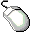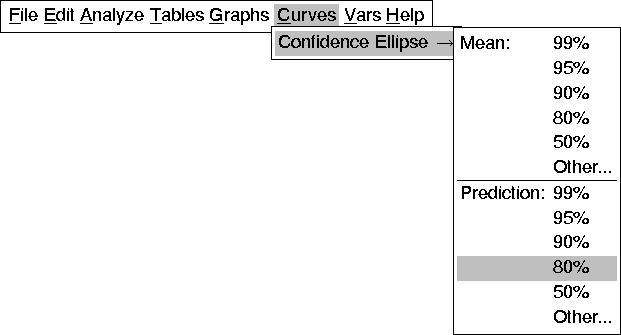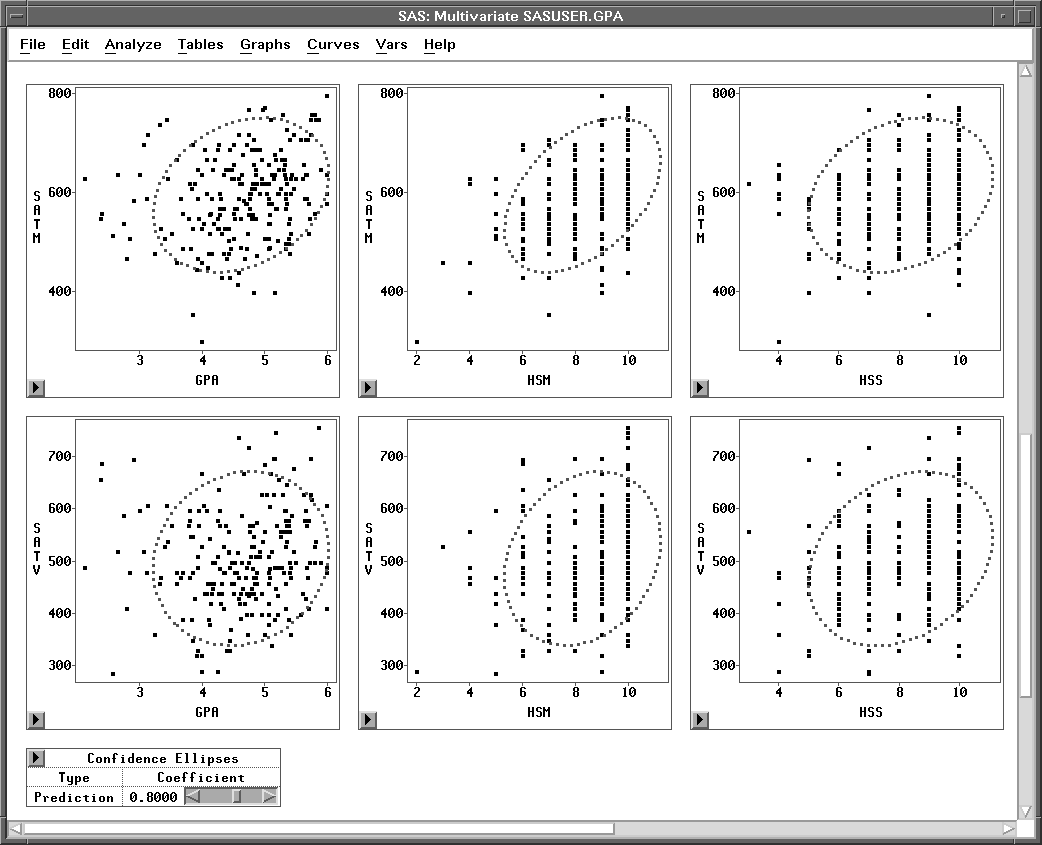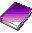Examining Correlations

## Confidence Ellipses

To learn more about correlations in the data, add a scatter plot matrix with confidence ellipses for all of the variables under consideration.Choose Curves:Confidence Ellipse:Prediction: 80%.Figure 18.6: Curves Menu

The lower half of the scatter plot matrix for the six variables appears on your display with the 80% prediction confidence ellipses drawn, as shown in Figure 18.7.Figure 18.7: Multivariate Window with Confidence Ellipses

There are two ways to interpret the ellipses: as confidence curves for bivariate normal distributions and as indicators of correlation.

As confidence curves, the ellipses show where the specified percentage of the data should lie, assuming a bivariate normal distribution. Under bivariate normality, the percentage of observations falling inside the ellipse should closely agree with the specified confidence level. You can examine the effect of increasing or decreasing the confidence level by adjusting the slider in the Confidence Ellipses table below the scatter plot matrix.

Confidence ellipses can also serve as visual indicators of correlations. The confidence ellipse collapses diagonally as the correlation between two variables approaches 1 or -1. The confidence ellipse is more circular when two variables are uncorrelated.

In this case the scatter plots for high school scores (HSM, HSS, and HSE) show a granular appearance that indicates the data are not continuous. These scatter plots clearly do not follow a bivariate normal distribution; therefore, it is not appropriate to interpret confidence ellipses.

The confidence ellipses for GPA, SATM, and SATV can be interpreted. These confidence ellipses contain observations appropriate to the 80% confidence level you specified. The nearly circular appearance of the confidence ellipse in the plot of GPA versus SATV reflects the small correlation you observed in the Correlation Matrix table. The ellipse in the plot of GPA versus SATM is somewhat more elongated, reflecting a higher correlation.Note Visual interpretation of correlations can be subjective because changes in scale affect your perception (Moore and McCabe 1989). When examining correlations, you should use correlation coefficients as well as confidence ellipses.Related Reading Correlation Coefficients, Confidence Ellipses,Chapter 40.